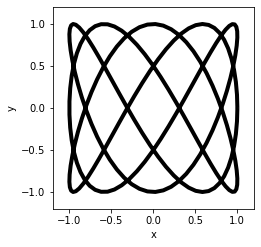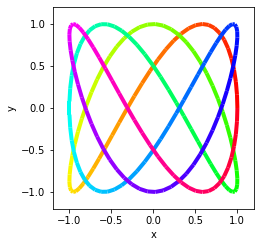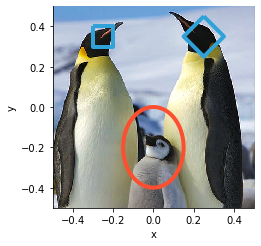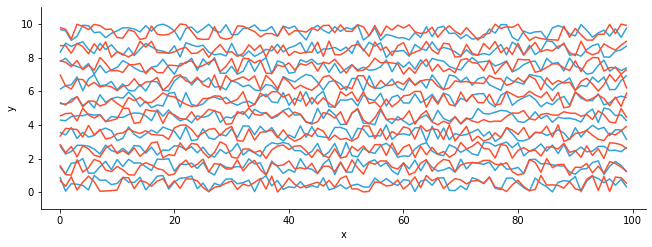# Path#

Title
Path Element
Dependencies
Matplotlib
Backends
Matplotlib
Bokeh
```import numpy as np
import holoviews as hv
from holoviews import opts

hv.extension('matplotlib')
```A `Path` element represents one more lines, connecting arbitrary points in two-dimensional space. `Path` supports plotting an individual line or multiple subpaths, which should be supplied as a list. Each path should be defined in a columnar format such as NumPy arrays, DataFrames or dictionaries for each column. For a full description of the path geometry data model see the Geometry Data User Guide.

In this example we will create a Lissajous curve, which describe complex harmonic motion:

```lin = np.linspace(0, np.pi*2, 200)

def lissajous(t, a, b, delta):
return (np.sin(a * t + delta), np.sin(b * t), t)

hv.Path([lissajous(lin, 3, 5, np.pi/2)]).opts(color='black', linewidth=4)
```If you looked carefully the `lissajous` function actually returns three columns, respectively for the x, y columns and a third column describing the point in time. By declaring a value dimension for that third column we can also color the Path by time. Since the value is cyclical we will also use a cyclic colormap (`'hsv'`) to represent this variable:

```hv.Path([lissajous(lin, 3, 5, np.pi/2)], vdims='time').opts(
cmap='hsv', color='time', linewidth=4)
```If we do not provide a `color` overlaid `Path` elements will cycle colors just like other elements do unlike `Curve` a single `Path` element can contain multiple lines that are disconnected from each other. A `Path` can therefore often useful to draw arbitrary annotations on top of an existing plot.

A `Path` Element accepts multiple formats for specifying the paths, the simplest of which is passing a list of `Nx2` arrays of the x- and y-coordinates, alternative we can pass lists of coordinates. In this example we will create some coordinates representing rectangles and ellipses annotating an `RGB` image:

```angle = np.linspace(0, 2*np.pi, 100)
baby = list(zip(0.15*np.sin(angle),  0.2*np.cos(angle)-0.2))

adultR = [(0.25, 0.45), (0.35,0.35), (0.25, 0.25), (0.15, 0.35), (0.25, 0.45)]
adultL = [(-0.3, 0.4), (-0.3, 0.3), (-0.2, 0.3), (-0.2, 0.4),(-0.3, 0.4)]

opts.Path(linewidth=4)
)
```A `Path` can also be used as a means to display a number of lines with the same sampling along the x-axis at once. If we initialize the `Path` with a tuple of x-coordinates and stacked y-coordinates, we can quickly view a number of lines at once. Here we will generate a number of random traces each slightly offset along the y-axis:

```N, NLINES = 100, 10
paths = hv.Path((np.arange(N), np.random.rand(N, NLINES) + np.arange(NLINES)[np.newaxis, :]))
paths2 = hv.Path((np.arange(N), np.random.rand(N, NLINES) + np.arange(NLINES)[np.newaxis, :]))

(paths * paths2).opts(aspect=3, fig_size=300)
```For full documentation and the available style and plot options, use `hv.help(hv.Path).`

This web page was generated from a Jupyter notebook and not all interactivity will work on this website. Right click to download and run locally for full Python-backed interactivity.